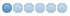# IAC GA (request)

• Topic Author
• Offline
• New Member
•2 years 2 months ago #8764 by Enceladus
IAC GA (request) was created by Enceladus
Has anyone had any luck implementing a telnet go ahead for their prompt? The client Mudlet basically requires prompts to contain IAC GA at the end or it will hang for a few hundred milliseconds before delivering the prompt. I did a simple fix by tacking on \xFF\xF9 after the > in this line in the make_prompt function in comm.c:

snprintf(prompt, sizeof(prompt), "%s> ", GET_NAME(d->character));

and thought everything looked groovy after, but then discovered that some things without a normal prompt don't function quite right, such as OLC.

Does anyone use Mudlet and have success with implementing IAC GA properly?

• Topic Author
• Offline
• New Member
•2 years 1 month ago #8773 by Enceladus
Replied by Enceladus on topic IAC GA (request)
Well here's what I did to make it work in the end ultimately. After limited testing, I couldn't find any bugs with it, though maybe snoop gets a little messed up. I borrowed a little bit of Luminari MUD's code for this as well. I'm just an amateur so if anyone has suggestions or advice let me know!

In comm.c find *make_prompt and replace as follows:
```static char *make_prompt(struct descriptor_data *d)
{
static char prompt[MAX_PROMPT_LENGTH];
size_t len = 0; /*moved these values up to here */
int count;
/* Note, prompt is truncated at MAX_PROMPT_LENGTH chars (structs.h) */

if (d->showstr_count)
{
/* # of pages to page through */
count = snprintf(prompt, sizeof(prompt),
"\t[ Return to continue, (q)uit, (r)efresh, (b)ack, or page number (%d/%d) ]\t",
d->showstr_page, d->showstr_count);
if (count >= 0)
len += count;
}
else if (d->str)
{                       /* for the modify-str system */
count = snprintf(prompt, sizeof(prompt), "] "); // strcpy: is NOT OK (for 'MAX_PROMPT_LENGTH >= 3')
if (count >= 0)
len += count;
} /* start building a prompt */
else if (STATE(d) == CON_PLAYING && !IS_NPC(d->character)) {

/*  *prompt = '\0';  */

if (GET_INVIS_LEV(d->character) && len < sizeof(prompt)) {
count = snprintf(prompt + len, sizeof(prompt) - len, "i%d ", GET_INVIS_LEV(d->character));
if (count >= 0)
len += count;
}
/* show only when below 25% */
if (PRF_FLAGGED(d->character, PRF_DISPAUTO) && len < sizeof(prompt)) {
struct char_data *ch = d->character;
if (GET_HIT(ch) << 2 < GET_MAX_HIT(ch) ) {
count = snprintf(prompt + len, sizeof(prompt) - len, "%dH ", GET_HIT(ch));
if (count >= 0)
len += count;
}
if (GET_MANA(ch) << 2 < GET_MAX_MANA(ch) && len < sizeof(prompt)) {
count = snprintf(prompt + len, sizeof(prompt) - len, "%dM ", GET_MANA(ch));
if (count >= 0)
len += count;
}
if (GET_MOVE(ch) << 2 < GET_MAX_MOVE(ch) && len < sizeof(prompt)) {
count = snprintf(prompt + len, sizeof(prompt) - len, "%dV ", GET_MOVE(ch));
if (count >= 0)
len += count;
}
} else { /* not auto prompt */
if (PRF_FLAGGED(d->character, PRF_DISPHP) && len < sizeof(prompt)) {
count = snprintf(prompt + len, sizeof(prompt) - len, "%dH ", GET_HIT(d->character));
if (count >= 0)
len += count;
}

if (PRF_FLAGGED(d->character, PRF_DISPMANA) && len < sizeof(prompt)) {
count = snprintf(prompt + len, sizeof(prompt) - len, "%dM ", GET_MANA(d->character));
if (count >= 0)
len += count;
}

if (PRF_FLAGGED(d->character, PRF_DISPMOVE) && len < sizeof(prompt)) {
count = snprintf(prompt + len, sizeof(prompt) - len, "%dV ", GET_MOVE(d->character));
if (count >= 0)
len += count;
}
}

if (PRF_FLAGGED(d->character, PRF_BUILDWALK) && len < sizeof(prompt)) {
count = snprintf(prompt + len, sizeof(prompt) - len, "BUILDWALKING ");
if (count >= 0)
len += count;
}

if (PRF_FLAGGED(d->character, PRF_AFK) && len < sizeof(prompt)) {
count = snprintf(prompt + len, sizeof(prompt) - len, "AFK ");
if (count >= 0)
len += count;
}

if (GET_LAST_NEWS(d->character) < newsmod)
{
count = snprintf(prompt + len, sizeof(prompt) - len, "(news) ");
if (count >= 0)
len += count;
}

if (GET_LAST_MOTD(d->character) < motdmod)
{
count = snprintf(prompt + len, sizeof(prompt) - len, "(motd) ");
if (count >= 0)
len += count;
}

if (len < sizeof(prompt))
{
count = snprintf(prompt + len, sizeof(prompt) - len, "> ");
if (count >= 0)
len += count;
}
}
/*START OF NPC PROMPT FOR SWITCHED PLAYERS*/
else if (STATE(d) == CON_PLAYING && IS_NPC(d->character))
{
count = snprintf(prompt + len, sizeof(prompt) - len, "%s>%c%c",GET_NAME(d->character),(char)IAC, (char)GA);
if (count >= 0)
len += count;
}
/*END OF NPC PROMPT FOR SWITCHED PLAYERS*/

else
*prompt = '\0';
if (len < sizeof(prompt))
{
count = snprintf(prompt + len, sizeof(prompt) - len, "%c%c",(char)IAC, (char)GA);
if (count >= 0)
len += count;
}

return (prompt);
}```

• thomas
•• Offline
•2 years 1 month ago #8775 by thomas
Replied by thomas on topic IAC GA (request)
Have you tested this with a regular telnet client as well?

•